# How to find the maximum value of Bitwise AND from root to leaf in a Binary tree?

## Introduction

Before jumping to the question let's first know what Bitwise AND operator is.

The bitwise AND (&) is a binary operation that gives binary representation 1 (1*1=1) if both the bits are 1 otherwise the result is 0 (0*0=0, 1*0=0).

## Problem statement

We are given a Binary tree. Our goal is to find the maximum Bitwise AND of all nodes in any path from the root to the leaf node.

Example:

Input1: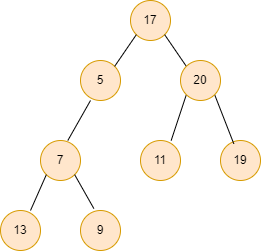Output1: 16

Explanation:

Path1: (17 & 5 & 7 & 13) = 1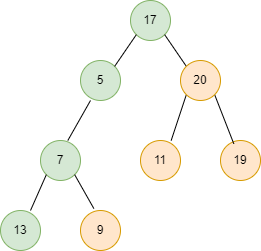Path2: (17 & 5 & 7 & 9) = 1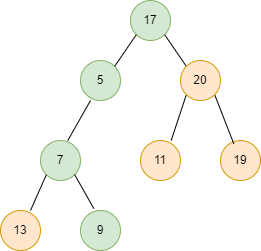Path3: (17 & 20 & 11) = 0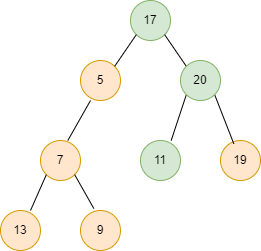Path4: (17 & 20 & 19) = 16  // maximum value of Bitwise AND from root to leaf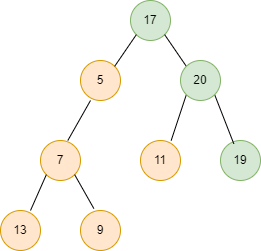Input 2: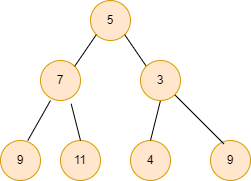Output2: 1

Explanation:

Path1: (5 & 7 & 9) = 1 // maximum value of Bitwise AND from root to leaf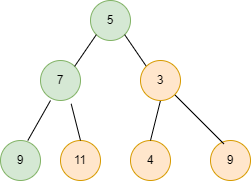## Approach

The idea is simple, traverse through every path from the root to leaf in the given binary tree and calculate the bitwise AND of all the nodes that occurred in the paths one by one. Finally, calculate the maximum of all these values.

Steps:

• Declare a global variable MAX_AND = 0, which stores the maximum value of Bitwise AND from root to leaf.
• Declare a curr_ans variable in the main function and initialise with root->data.
• Now, inside the maximum_AND function, if root=NULL return.
• Else check if it is a leaf node then do curr_ans = (curr_ans & root->data), take maximum of curr_ans and MAX_AND and return.
• Else call recursively for the left and right subtree and replace the curr_ans parameter from (curr_ans & root->data).

Let’s understand the above approach with an example:

Input: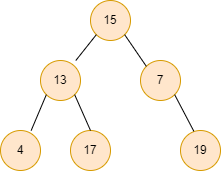So, initially MAX_AND = 0, curr_ans = root->data. We start traversing all the paths one by one. If we reach any leaf node, we calculate the Bitwise AND of all the path nodes and take the maximum from all the paths.

• MAX_AND = 0, curr_ans = 15 // root data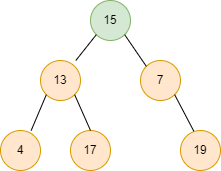• MAX_AND = 0, curr_ans = (15 & 13)=13.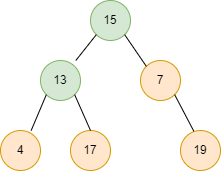• Now, we encountered a leaf node, curr_ans = (15 & 13 & 4) = 4, MAX_AND = max(4, 0) = 4.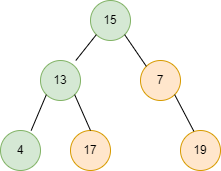• Again, we encountered a leaf node, curr_ans = (15 & 13 & 17) = 1, MAX_AND = max(1, 4) = 4.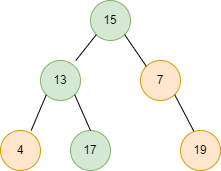• MAX_AND = 4, curr_ans = ( 15 & 7) = 7.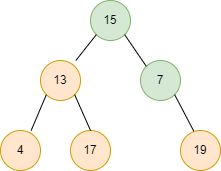• We encountered a leaf node, curr_ans = ( 15 & 7 & 19) = 3, MAX_AND = (3,4) = 4.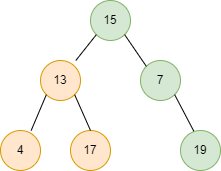Finally, print the MAX_AND = 4which is the maximum value of Bitwise AND from root to leaf.

## Code in C++

``````#include <bits/stdc++.h>
using namespace std;

int MAX_AND = 0;

struct Node {
int data;

Node *left, *right;

Node(int x)
{
data = x;
left = NULL;
right = NULL;
}
};

void maximum_AND(Node* root, int curr_ans)
{

if (root == NULL)
return;

if (root->left == NULL
&& root->right == NULL) {
curr_ans = curr_ans & root->data;

MAX_AND = max(curr_ans, MAX_AND);

return;
}

maximum_AND(root->left,
curr_ans & root->data);

maximum_AND(root->right,
curr_ans & root->data);
}

int main()
{

Node* root = new Node(17);
root->left = new Node(5);
root->right = new Node(20);
root->left->left = new Node(7);
root->left->left->left = new Node(13);
root->left->left->right = new Node(7);
root->right->left = new Node(11);
root->right->right = new Node(19);

maximum_AND(root, root->data);

cout << MAX_AND << endl;

return 0;
}``````

Output

``16``

### Complexity Analysis

Time complexity: O(n), where n is the number of nodes in the given tree as we are traversing all the nodes.

Space complexity: O(h), where h is the height of the given binary tree.

Q1. In a binary tree, what is the maximum number of leaves?

Ans: The maximum number of leaves in a node binary tree is 2^(h+1)-1, where h is the tree's height. The more leaf nodes a tree has, the more balanced it is. A tree that is completely skewed to one side will only have one leaf. As an example, if you have a fully balanced tree with n nodes, the height will be (log 2n), and the number of leaves will be 2^(log2n - 1).

Q2. What is the difference between logical AND and Bitwise AND?

Ans: The logical AND operator returns only Boolean values when applied to Boolean expressions. The bitwise AND operator accepts and returns data of the types integer, short int, long, and unsigned int.

Q3. How is stack used in memory management?

Ans: A stack is a memory section in a computer that keeps temporary variables generated by a function. Variables are declared, stored, and initialised in the stack during runtime. It's a type of transient memory. The memory of the variable will be automatically wiped once the computational process is completed.

## Key Takeaways

So, this article discussed the Bitwise AND(&) and the problem of finding the maximum value of Bitwise AND from root to leaf in a Binary tree with examples for better understanding and its implementation in C++.

If you are a beginner, interested in coding and want to learn DSA, you can look for our guided path for DSA, which is free!